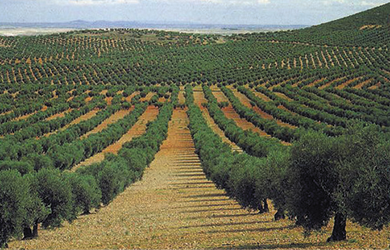# Calculations for the use of fertilizer solutionsDefinitions

Below we present some examples to show how fertilizer dosage is calculated whenever recommendations are available.

Recommendations are published in the following form:

 For nitrogen (N): 1 unit N = 1 Kg (pure) N For phosphorus (P2O5): 1 unit = 1 Kg P2O5 For potassium (K2O): 1 unit = 1 Kg K2O

All recommendations should be carefully studied and evaluated. Since some recommendations are in terms of pure P and K instead of the oxides.

Calculation of fertilizer dosage

Example for field crops fertilization

The following fertilization recommendation has been given a farmer growing a drip- irrigated squash crop:

Basic dressing (as pre-transplant application) of 60 Kg N, 120 Kg P2O5 and 70 Kg K2O per hectare.

Top dressing during the 10 mid-season weeks 210 Kg of N, 70 Kg of P2O5 and 210 Kg of K2O should be fertigated per hectare.

Calculating the pre-plant application

The recommended rates are 60 – N; 120 – P2O5 and 70 – K2O, given ratio of 1:2:1.2.

For this recommendation “Gatit 14-28-18+TE” fertilizer is the best approximation to the above recommendation. The following amount of “Gatit 14-28-18+TE” is required:

 60Kg N / ha × 100 = 430 Kg “Gatit 14-28-18+TE” / ha 14

This quantity supplies the required amounts of N, P2O5 and K2O.

Calculating the mid-season application

The recommended rates are 210 – N, 70 – P2O5 , 210 – K2O , given ratio of 3:1:3 “Gatit 21-7-21+TE” fertilizer fits the recommendation.

The required amount of fertilizer is:

 210Kg N / ha × 100 = 1000 Kg “Gatit 21-7-21+TE” / ha 21

A total amount of 1000 kg of fertilizer should be applied during a period of ten weeks. Therefore, every week:

 1000Kg “Gatit 21-7-21+TE” / ha × 100 = 100 Kg “Gatit 21-7-21+TE” / ha / week 21

should be applied

A second alternative is to inject the fertilizer solution at constant concentration into the irrigation water (proportional fertigation)

If during that 10 week period, a total of 3000 m³ water/ha are to be applied, then the following “proportional” dosage should be maintained:

 1000Kg “Gatit 21-7-21+TE” / ha × 100 = 0.330 Kg “Gatit 21-7-21+TE” / m³ 3000m3water / ha

Example for greenhouse fertigation

The following recommendation has been given to a grower of greenhouse carnations: Nitrogen (N): 120 ppm; Phosphorus (P2O5): 60 ppm; Potassium (K2O): 240 ppm. The relationship between the three nutrients is: 2:1:4.
Gat fertilizers “Shafir4-2-8” has the adequate ratio of nutrients.

The following calculations are performed:
To reach a concentration of 1 ppm N, 1 gram N should be added to each cubic meter of irrigation water, (1 gr N per 1,000,000 gr water = 1 gr N /m³). Therefore, in order to reach 120 ppm, 120 gr N (0.12 Kg N) should be added to each m³ of irrigation water.
The required weight of “Shafir4-2-8” is calculated as follows:

 0.2Kg N / m³ × 100 = 3 Kg “Shafir 4-2-8” / m³ 4

The volume weight of “Shafir4-2-8” is 1.17 gr/cm³ (=1.17 Kg per Lt)

Therefore, the volume of fertilizer solution to be injected into the irrigation water is:

 3Kg “Shafir 4-2-8” / m³ = 2.56 Lt “Shafir 4-2-8” / m³ 1.17Kg / Lt